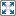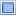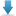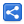# Groovy Operators - Spread Operator

[Last Updated: Dec 18, 2018]

The spread-dot operator (*.) is used to invoke an action on all items of an aggregate object.

It is equivalent to calling the action on each item and collecting the result into a list.

# Examples

## Calling method and collecting returned values

In following method we are going to call size() method of each nested list via spread operator:

#### src/Example1Simple.groovy

```def lists = [, [10, 20, 30], [6, 8]]
def sizes = lists*.size()
println sizes```

#### Output

`[1, 3, 2]`

## Collecting properties

#### src/Example2Properties.groovy

```class Person{
String name;
int age;

Person(String name, int age) {
this.name = name
this.age = age
}
}
def persons = [new Person("Tina", 31), new Person("Sam", 41)];

def names = persons*.name
println names

def ages = persons.age
println ages

//nested
def lengths = persons*.name*.length()
println lengths```

#### Output

`[Tina, Sam][31, 41][4, 3]`

## Calling method with arguments

#### src/Example3Calls.groovy

```def bds = [BigDecimal.valueOf(12), BigDecimal.valueOf(1.1)]
println sums```

#### Output

`[22, 11.1]`

In following example we are calling getClass() and then getName() (Check out direct field access operator):

#### src/Example4Reflection.groovy

```def bds = [BigDecimal.ONE, BigInteger.TEN]
def classNames = bds*.class.name
println classNames

```

#### Output

`[java.math.BigDecimal, java.math.BigInteger]`

## Using any Iterable

The spread operator can be used on any class which implements the `Iterable` interface. In following example shows spread operator with `Path` which implements `Iterable`

#### src/Example5Iterable.groovy

```import java.nio.file.Path
import java.nio.file.Paths

def names = path*.getFileName()
println names

```

#### Output

`[home, joe, app, readMe.txt]`

## Null safety

The spread operator is null-safe, meaning that if an element of the collection is null, it will return null instead of throwing a `NullPointerException`:

#### src/Example6NullSafe.groovy

```def lists = [null, [10, 20, 30], [6, 8]]
def sizes = lists*.size()
println sizes

```

#### Output

`[null, 3, 2]`

An iterable can be expanded into individual method arguments via spread operator (only *, no dot):

```def sum(int x, int y, int z) {
return x + y + z;
}

def numbers = [1, 3, 5]
def sum = sum(*numbers);
println sum

```

We can also use spread operator to pass a list to a method with array parameter:

#### src/Example8MethodArgs2.groovy

```def sum(int[] args) {
def result = 0;
for (int i = 0; i < args.length; i++) {
result += args[i]
}
return result;
}

def numbers = [1, 3, 5]
def sum = sum(*numbers);
println sum
```

#### Output

`9`

In above example, if we don't use spread operator then an exception will be thrown

```def sum(int[] args) {
def result = 0;
for (int i = 0; i < args.length; i++) {
result += args[i]
}
return result;
}

def numbers = [1, 3, 5]
def sum = sum(numbers);```
```Cannot cast object '[1, 3, 5]' with class 'java.util.ArrayList' to class 'java.lang.Integer'
```

When spread operator (*) used inside a list literal, the elements are inlined into the list:

#### src/Example9ListElements.groovy

```def numbers = [1,2,3]
def numbers2 = [0, *numbers, 4,5,6]
println numbers2```

#### Output

`[0, 1, 2, 3, 4, 5, 6]`

The spread map operator (*:) works in a similar manner as the spread list operator

#### src/Example9MapElements.groovy

```def map = [x: 1, y: 2]
def map2 = [a: 0, *:map, z: 3]
println map2```

#### Output

`[a:0, x:1, y:2, z:3]`

## Example Project

Dependencies and Technologies Used:

• Groovy 2.5.3
• JDK 9.0.1
 Groovy - Spread OperatorSelect AllDownloadShare